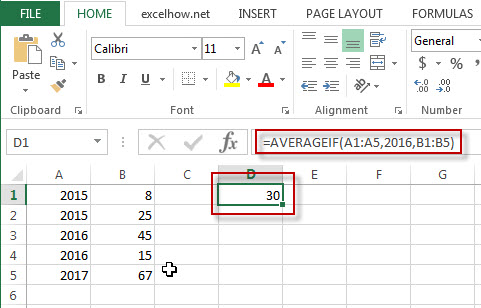## Excel AverageIF Function

This post will guide you how to use Excel AVERAGEIF function with syntax and examples in Microsoft excel.

### Description

The Excel AVERAGEAIF function returns the average of all numbers in a range of cells that meet a given criteria.

The AVERAGEIF function is a build-in function in Microsoft Excel and it is categorized as a Statistical Function.

The AVERAGEIF function is available in Excel 2016, Excel 2013, Excel 2010, Excel 2007, Excel 2003, Excel XP, Excel 2000, Excel 2011 for Mac.

### Syntax

The syntax of the AVERAGEIF function is as below:

`= AVERAGEIF (range, criteria, [average_range])`

Where the AVERAGEIF function arguments are:

• Range -This is a required argument.  The range of cells that you want to apply the criteria to average
• Criteria – This is a required argument.  The criteria used to define which cells are averaged
• Average_range – This is an optional argument.  The average range is actually to average. If it is omitted, the function will use Range values to average.

### Example

The below examples will show you how to use Excel AVERAGEIF Function to return the average of all numbers in a range of cells based on a given criteria.

#1 To get the average of all numbers in average range B1:B5 and meet the criteria that equal to “2016” in range A1:A5, and the, just using the following excel formula: =AVERAGEIF(A1:A5,2015,B1:B5)